Starts With A Bang

# Ask Ethan: Is There A Fundamental Reason Why E = mc²?

#### Physics demands that it couldn’t be any other way. Here’s why.

Ask anyone — even someone with no background in science — to name something that Einstein did, and odds are they’ll come back with his most famous equation: E = mc². In plain English, it tells us that energy is equal to mass multiplied by the speed of light squared, teaching us an enormous amount about the Universe. This one equation tells us how much energy is inherent to a massive particle at rest, and also tells us how much energy is required to create particles (and antiparticles) out of pure energy. It tells us how much energy is released in nuclear reactions, and how much energy comes out of annihilations between matter and antimatter.

But why? Why does energy have to equal mass multiplied by the speed of light squared? Why couldn’t it have been any other way? That’s what Brad Stuart wants to know, writing in to ask:

“Einstein’s equation is amazingly elegant. But is its simplicity real or only apparent? Does E = mc² derive directly from an inherent equivalence between any mass’s energy and the square of the speed of light (which seems like a marvelous coincidence)? Or does the equation only exist because its terms are defined in a (conveniently) particular way?”

It’s a great question. Let’s investigate Einstein’s most famous equation, and see exactly why it couldn’t have been any other way.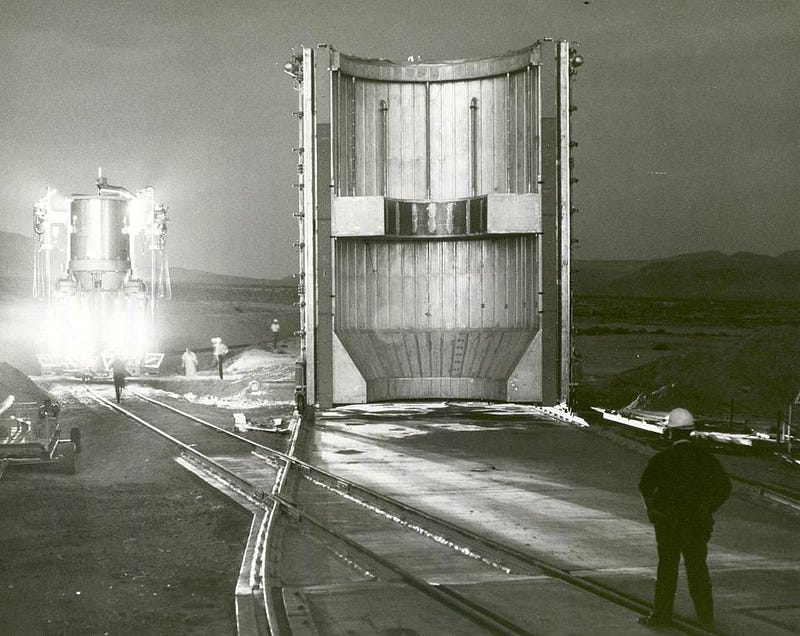A nuclear-powered rocket engine, preparing for testing in 1967. This rocket is powered by Mass/Energy conversion, and is underpinned by the famous equation E=mc². (ECF (EXPERIMENTAL ENGINE COLD FLOW) EXPERIMENTAL NUCLEAR ROCKET ENGINE, NASA, 1967)

To start with, it’s important to realize a few things about energy. Energy, especially to a non-physicist, is a particularly tricky thing to define. There are many examples we can all come up with off the tops of our heads.

• There’s potential energy, which is some form of stored energy that can be released. Examples include gravitational potential energy, like lifting a mass up to a large height, chemical potential energy, where stored energy in molecules like sugars can undergo combustion and be released, or electric potential energy, where built-up charges in a battery or capacitor can be discharged, releasing energy.
• There’s kinetic energy, or the energy inherent to a moving object due to its motion.
• There’s electrical energy, which is the kinetic energy inherent to moving charges and electrical currents.
• There’s nuclear energy, or the energy released by nuclear transitions to more stable states.

And, of course, there are many other types. Energy is one of those things that we all “know it when we see it,” but to a physicist, we want a more universal definition. The best one we have is simply: extracted/extractable energy is a way of quantifying our ability to perform work.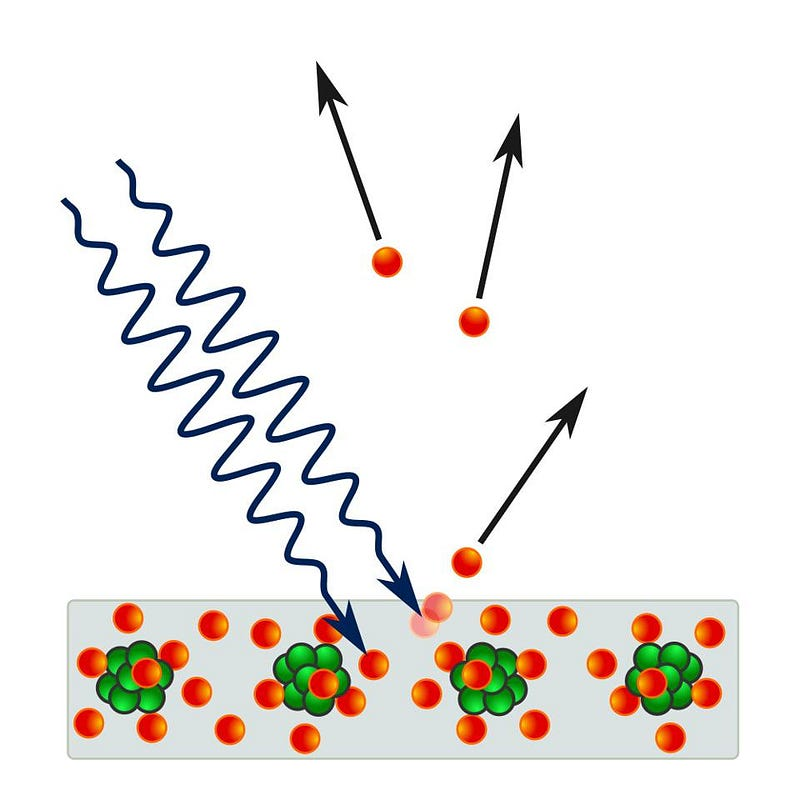The photoelectric effect details how electrons can be ionized by photons based on the wavelength of individual photons, not on light intensity or total energy or any other property. If a quantum of light comes in with enough energy, it can interact with and ionize an electron, kicking it out of the material and leading to a detectable signal. These photons carry energy, and perform work on the electrons that they strike. (PONOR / WIKIMEDIA COMMONS)

Work, to a physicist, has a particular definition itself: a force exerted in the same direction that an object is moved, multiplied by the distance the object moves in that direction. Lifting a barbell up to a certain height does work against the force of gravity, raising your gravitational potential energy; releasing that raised barbell converts that gravitational potential energy into kinetic energy; the barbell striking the floor converts that kinetic energy into a combination of heat, mechanical, and sound energy. Energy isn’t created or destroyed in any of these processes, but rather converted from one form into another.

The way most people think about E = mc², when they first learn about it, is in terms of what we call “dimensional analysis.” They say, “okay, energy is measured in Joules, and a Joule is a kilogram · meter² per second². So if we want to turn mass into energy, you just need to multiply those kilograms by something that’s a meter² per second², or a (meter/second)², and there’s a fundamental constant that comes with units of meters/second: the speed of light, or c.” It’s a reasonable thing to think, but that’s not enough.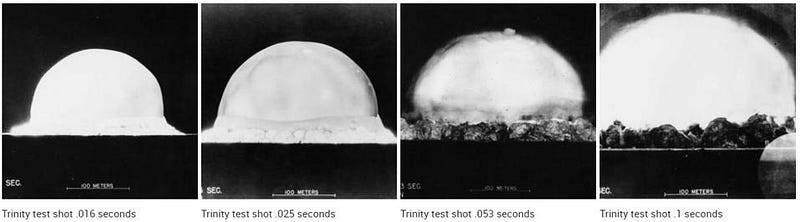These four panels show the Trinity test explosion, the world’s first nuclear (fission) bomb, at a respective 16, 25, 53, and 100 milliseconds after ignition. The highest temperatures come in the earliest moments of ignition, before the volume of the explosion dramatically increases. (ATOMIC HERITAGE FOUNDATION)

After all, you can measure any velocity you want in units of meters/second, not just the speed of light. In addition, there’s nothing preventing nature from requiring a proportionality constant — a multiplicative factor like ½, ¾, 2π, etc. — to make the equation true. If we want to understand why the equation must be E = mc², and why no other possibilities are allowed, we have to imagine a physical situation that could tell the difference between various interpretations. This theoretical tool, known as a gedankenexperiment or thought-experiment, was one of the great ideas that Einstein brought from his own head into the scientific mainstream.

What we can do is imagine that there’s some energy inherent to a particle due to its rest mass, and additional energy that it might have due to its motion: kinetic energy. We can imagine starting a particle off high up in a gravitational field, as though it started off with a large amount of gravitational potential energy, but at rest. When you drop it, that potential energy converts into kinetic energy, while the rest mass energy stays the same. At the moment just prior to impact with the ground, there will be no potential energy left: just kinetic energy and the energy inherent to its rest mass, whatever that may be.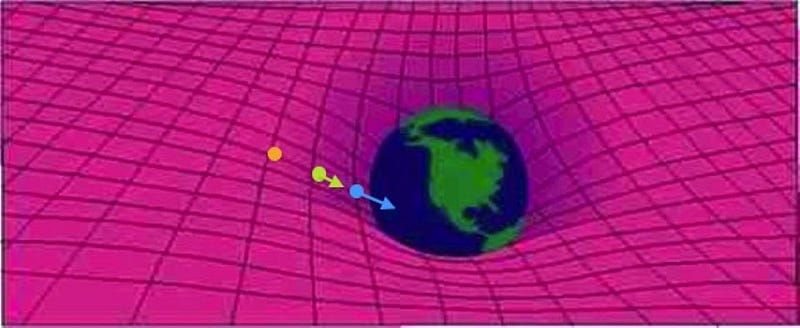If you have a particle (or a particle-antiparticle pair) at rest above the surface of the Earth, in orange, it will have no kinetic energy but lots of potential energy. If the particle or system is then released and allowed to fall freely, it will gain kinetic energy as the potential energy is transformed into the energy of motion. This thought experiment is one way to demonstrate the insufficiency of special relativity. (RAY SHAPP / MIKE LUCIUK; E. SIEGEL)

Now, with that picture in our heads — that there’s some energy inherent to the rest mass of a particle and that gravitational potential energy can be converted into kinetic energy (and vice versa) — let’s throw in one more idea: that all particles have an antiparticle counterpart, and if ever the two of them collide, they can annihilate away into pure energy.

(Sure, E = mc² tells us the relationship between mass and energy, including how much energy you need to create particle-antiparticle pairs out of nothing, and how much energy you get out when particle-antiparticle pairs annihilate. But we don’t know that yet; we want to establish this must be the case!)

So let’s imagine, now, that instead of having one particle high up in a gravitational field, imagine that we have both a particle and an antiparticle up high in a gravitational field, ready to fall. Let’s set up two different scenarios for what could happen, and explore the consequences of both.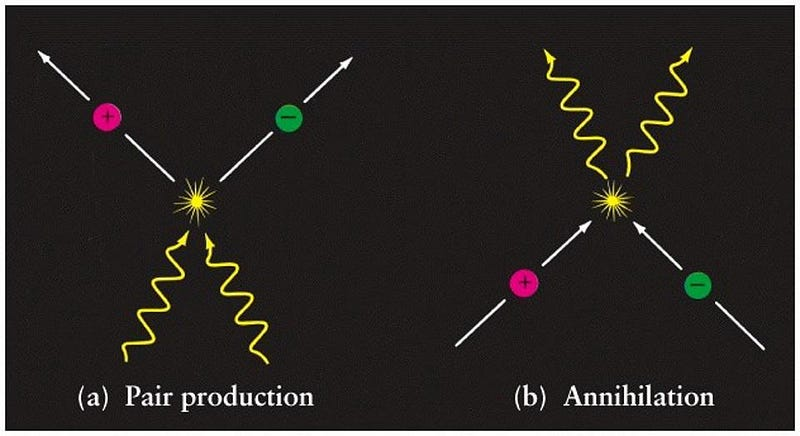The production of matter/antimatter pairs (left) from pure energy is a completely reversible reaction (right), with matter/antimatter annihilating back to pure energy. For many particle systems, however, reversibility is not guaranteed. (DMITRI POGOSYAN / UNIVERSITY OF ALBERTA)

Scenario 1: the particle and antiparticle both fall, and annihilate at the instant they would hit the ground. This is the same situation we just thought about, except doubled. Both the particle and antiparticle start with some amount of rest-mass energy. We don’t need to know the amount, simply that’s whatever that amount is, it’s equal for the particle and the antiparticle, since all particles have identical masses to their antiparticle counterparts.

Now, they both fall, converting their gravitational potential energy into kinetic energy, which is in addition to their rest-mass energy. Just as was the case before, the instant before they hit the ground, all of their energy is in just two forms: their rest-mass energy and their kinetic energy. Only, this time, just at the moment of impact, they annihilate, transforming into two photons whose combined energy must equal whatever that rest-mass energy plus that kinetic energy was for both the particle and antiparticle.

For a photon, however, which has no mass, the energy is simply given by its momentum multiplied by the speed of light: E = pc. Whatever the energy of both particles was before they hit the ground, the energy of those photons must equal that same total value.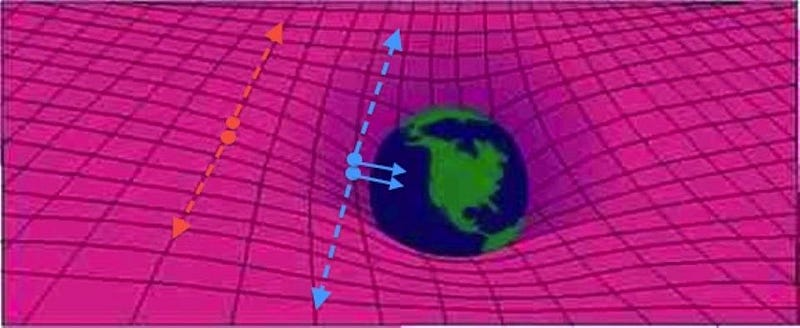If you were to annihilate a particle-antiparticle pair into pure energy (two photons) with a lot of gravitational potential energy, only the rest mass energy (orange) gets converted into photon energy. If you were to drop that particle and antiparticle towards Earth’s surface, and only allowed them to annihilate just before impact, they’d have significantly more energy and produce bluer, more energetic photons. (RAY SHAPP / MIKE LUCIUK; E. SIEGEL)

Scenario 2: the particle and antiparticle both annihilate into pure energy, and then fall the rest of the way down to the ground as photons, with zero rest mass. Now, let’s imagine an almost identical scenario. We start with the same particle and antiparticle, high up in a gravitational field. Only, this time, when we “release” them and allow them to fall, they annihilate into photons immediately: the entirety of their rest-mass energy gets turned into the energy of those photons.

Because of what we learned before, that means the total energy of those photons, where each one has an energy of E = pc, must equal the combined rest-mass energy of the particle and antiparticle in question.

Now, let’s imagine that those photons eventually make their way down to the surface of the world that they’re falling onto, and we measure their energies when they reach the ground. By the conservation of energy, they must have a total energy that equals the energy of the photons from the previous scenario. This proves that photons must gain energy as they fall in a gravitational field, leading to what we know as a gravitational blueshift, but it also leads to something spectacular: the notion that E = mc² is what a particle’s (or antiparticle’s) rest mass has to be.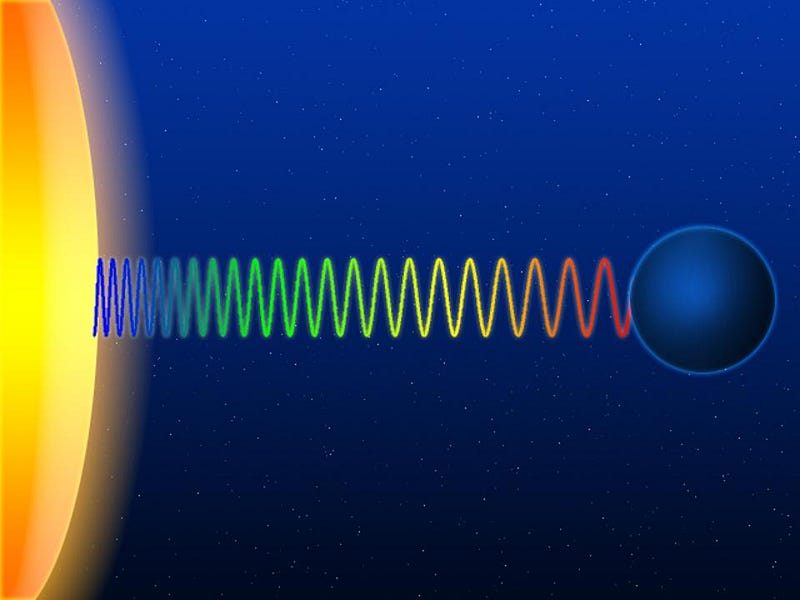When a quantum of radiation leaves a gravitational field, its frequency must be redshifted to conserve energy; when it falls in, it must be blueshifted. Only if gravitation itself is linked to not only mass but energy, too, does this make sense. Gravitational redshift is one of the core predictions of Einstein’s General Relativity, but has only recently been tested directly in such a strong-field environment as our galactic center. (VLAD2I AND MAPOS / ENGLISH WIKIPEDIA)

There’s only one definition of energy we can use that universally applies to all particles — massive and massless, alike — that enables scenario #1 and scenario #2 to give us identical answers: E = √(m²c⁴ + p²c²). Think about what happens here under a variety of conditions.

• If you are a massive particle at rest, with no momentum, your energy is just √(m²c⁴), which becomes E = mc².
• If you’re a massless particle, you must be in motion, and your rest mass is zero, so your energy is just √(p²c²), or E = pc.
• If you’re a massive particle and you’re moving slow compared to the speed of light, then you can approximate your momentum by p = mv, and so your energy becomes √(m²c⁴ + m²v²c²). You can rewrite this as E = mc² · √(1 + v²/c²), so long as v is small compared to the speed of light.

If you don’t recognize that last term, don’t worry. You can perform what’s known, mathematically, as a Taylor series expansion, where the second term in parentheses is small compared to the “1” that makes up the first term. If you do, you’ll get that E = mc² · [1 + ½(v²/c²) + …], where if you multiply through for the first two terms, you get E = mc² + ½mv²: the rest mass plus the old-school, non-relativistic formula for kinetic energy.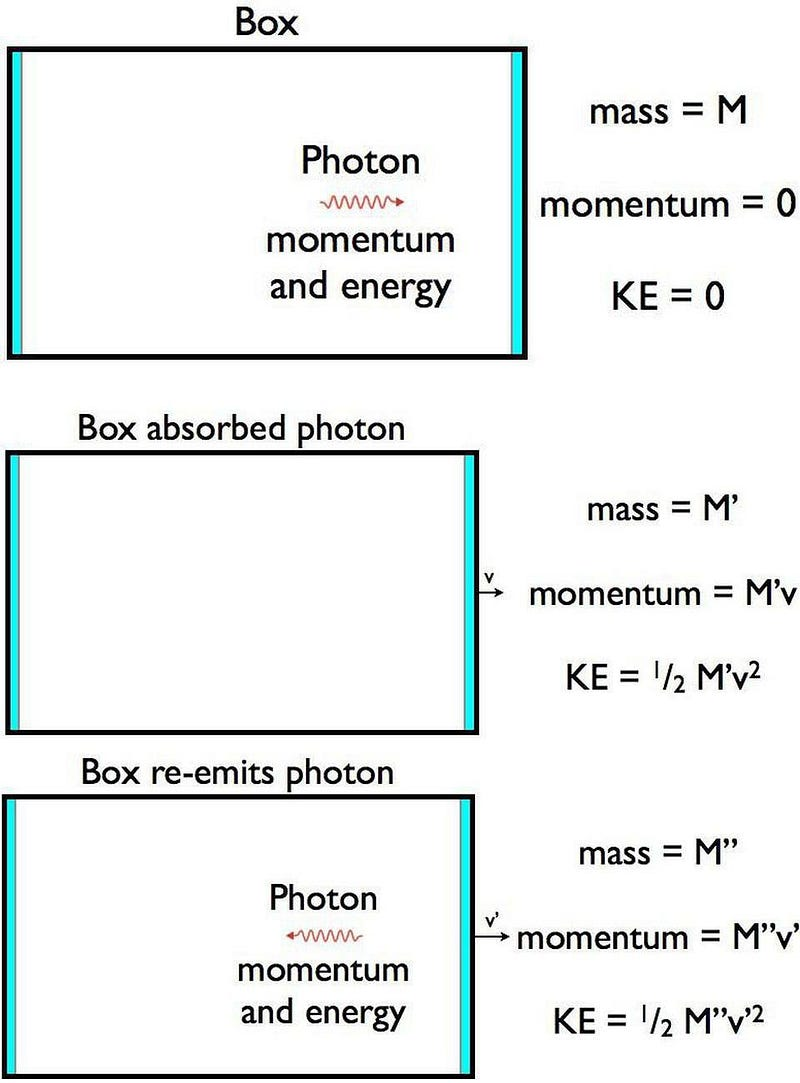A photon traveling in a box, striking the box, and getting re-emitted in the opposite direction is enough of a setup, along with the stipulation that energy and momentum must both be conserved, to derive Einstein’s most famous equation: E = mc². (E. SIEGEL)

This is absolutely not the only way to derive E = mc², but it is my favorite way to look at the problem. Three other ways can be found three here, here and here, with some good background here on how Einstein originally did it himself. If I had to choose my second favorite way to derive that E = mc² for a massive particle at rest, it would be to consider a photon — which always carries energy and momentum — traveling in a stationary box with a mirror on the end that it’s traveling towards.

When the photon strikes the mirror, it temporarily gets absorbed, and the box (with the absorbed photon) has to gain a little bit of energy and start moving in the direction that the photon was moving: the only way to conserve both energy and momentum.

When the photon gets re-emitted, it’s moving in the opposite direction, and so the box (having lost a little mass from re-emitting that photon) has to move forward a little more quickly in order to conserve energy and momentum.

By considering these three steps, even though there are a lot of unknowns, there are a lot of equations that have to always match up: between all three scenarios, the total energy and the total momentum must be equivalent. If you solve those equations, there’s only one definition of rest-mass energy that works out: E = mc².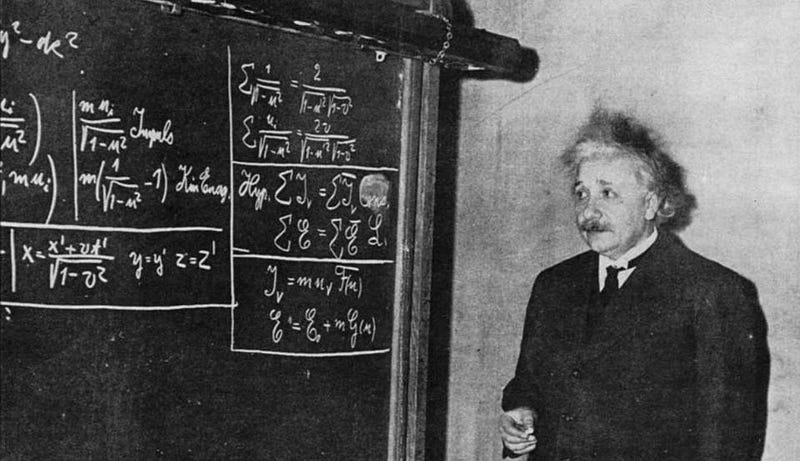Einstein deriving special relativity, for an audience of onlookers, in 1934. The consequences of applying relativity to the right systems require that, if we demand energy conservation, E = mc² must be valid. (PUBLIC DOMAIN IMAGE)

You can imagine that the Universe could have been very different from the one we inhabit. Perhaps energy didn’t need to be conserved; if this were the case, E = mc² wouldn’t need to be a universal formula for rest mass. Perhaps we could violate the conservation of momentum; if so, our definition for total energy — E = √(m²c⁴ + p²c²) — would no longer be valid. And if General Relativity weren’t our theory of gravity, or if a photon’s momentum and energy weren’t related by E = pc, then E = mc² wouldn’t be a universal relationship for massive particles.Travel the Universe with astrophysicist Ethan Siegel. Subscribers will get the newsletter every Saturday. All aboard!

But in our Universe, energy is conserved, momentum is conserved, and General Relativity is our theory of gravitation. Given these facts, all one needs to do is think of the proper experimental setup. Even without physically performing the experiment for yourself and measuring the outcomes, you can derive the one self-consistent answer for the rest-mass energy of a particle: only E = mc² does the job. We can try to imagine a Universe where energy and mass have some other relationship, but it would look very different from our own. It’s not merely a convenient definition; it’s the only way to conserve energy and momentum with the laws of physics that we have.

Send in your Ask Ethan questions to startswithabang at gmail dot com!

Starts With A Bang is written by Ethan Siegel, Ph.D., author of Beyond The Galaxy, and Treknology: The Science of Star Trek from Tricorders to Warp Drive.

Related
More work is needed before declaring the technique a fountain of youth.
Hinduism emphasizes the journey, whichever path that takes. And it holds us responsible for our own self-improvement.
An unexpected ancient manufacturing strategy may hold the key to designing concrete that lasts for millennia.
Quantum superposition challenges our notions of what is real.
The benefits of employee training are felt far and wide, from improvements in workers’ wellbeing to better customer interactions.

Up Next
The simulation hypothesis is fun to talk about, but believing it requires an act of faith.